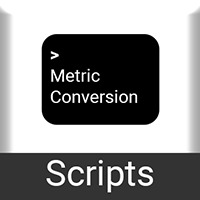# Metric Conversion Scripts

#### Chris Burgess

You must be logged in to obtain assets

### Description

Make any metric-imperial conversion easy with these 100+ scripts.

This asset includes help documentation if you get stuck. Below is a full list of currently available conversions (Note, you can convert between any two units on a given line. Example, Milliliters and Kilometers, or Centimeters and Inches)

Milli <-> Centi <-> Deci <-> Base <-> Deca <-> Hecto <-> Kilo (Meters, Liters, & Grams)

Acres <-> Hectares

Celsius <-> Farenheit

Mm<-> Cm<-> M<-> In <-> Ft <-> Yds <-> Mi<-> Km

Fluid Ounces <-> Cups <-> Pints <-> Quarts <-> Gallons <-> Liters

Ounces (solid) <-> Grams

Kilograms <-> Pounds <-> Short Tons (Tons) <-> Long Tons <-> Metric Tons

Square Ft <-> Square Meters <-> Square Kilometers <-> Square Miles <-> Square Yards